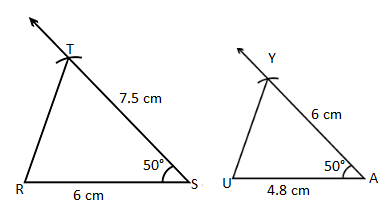# Maharashtra (MSBSHSE) SSC Board Question Paper For Class 10 Maths Paper 1 (Geometry) 2015 In PDF

## Maharashtra SSC Maths Geometry (Paper 1) Exam Question Paper 2015 with Solutions – Free Download

MSBSHSE (Maharashtra board) SSC Class 10 maths 2015 Geometry Question Paper with solutions are provided at BYJU’S, along with a downloadable pdf. Students are advised to prepare for the SSC Maths exam of Maharashtra board by dividing the syllabus into two parts, namely Geometry and Algebra. They can also get the two papers of MSBSHSE for each year, along with the stepwise solutions at BYJU’S. Students can access as well as download Msbshse Board SSC Maths previous years question papers at BYJU’s. Students can get full marks in class 10 Maths exam by practising all the important questions thoroughly. Memorising formulas of class 10 Maths will help the students in solving short answer questions quickly.

### MSBSHSE Class 10 Mathematics Question Paper 2015 Geometry Paper with Solutions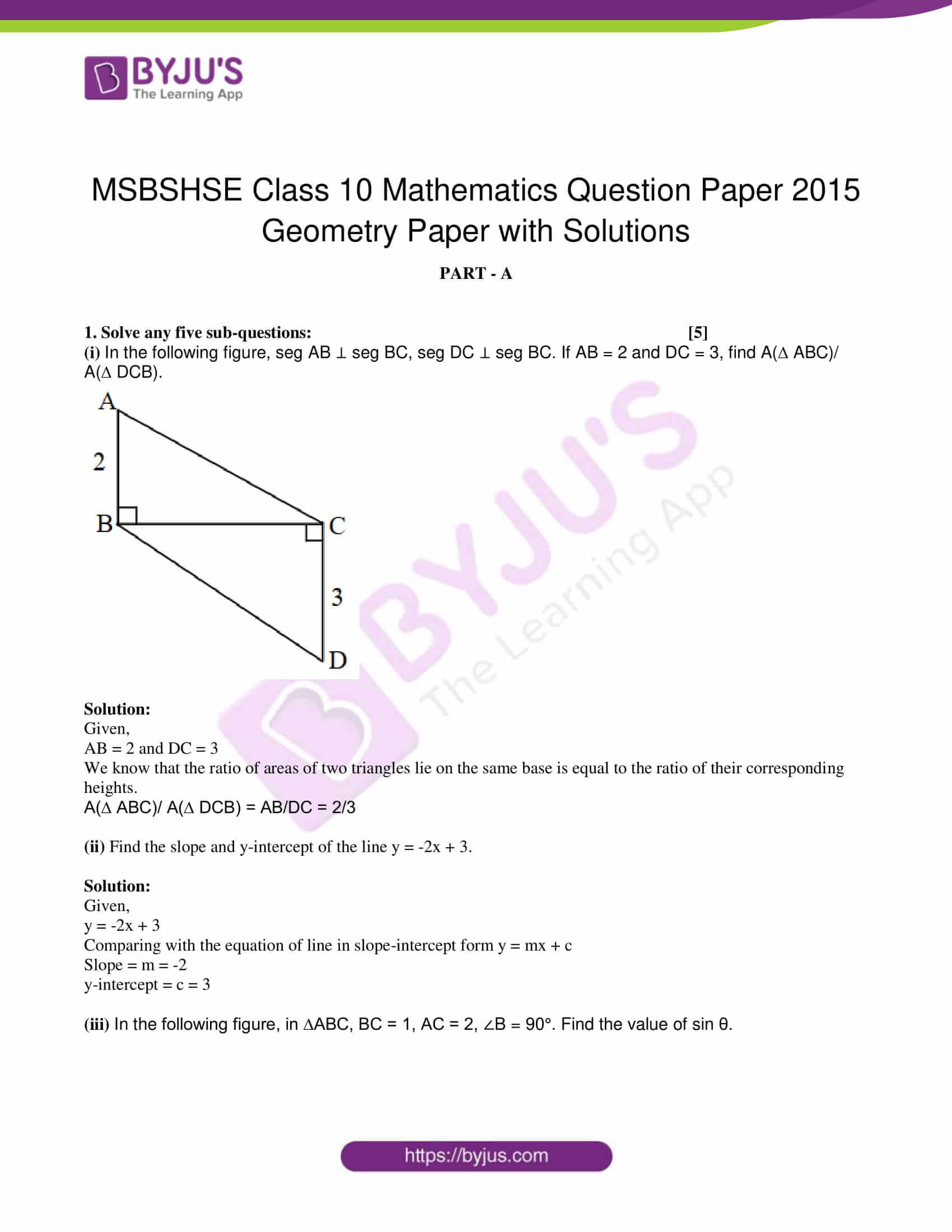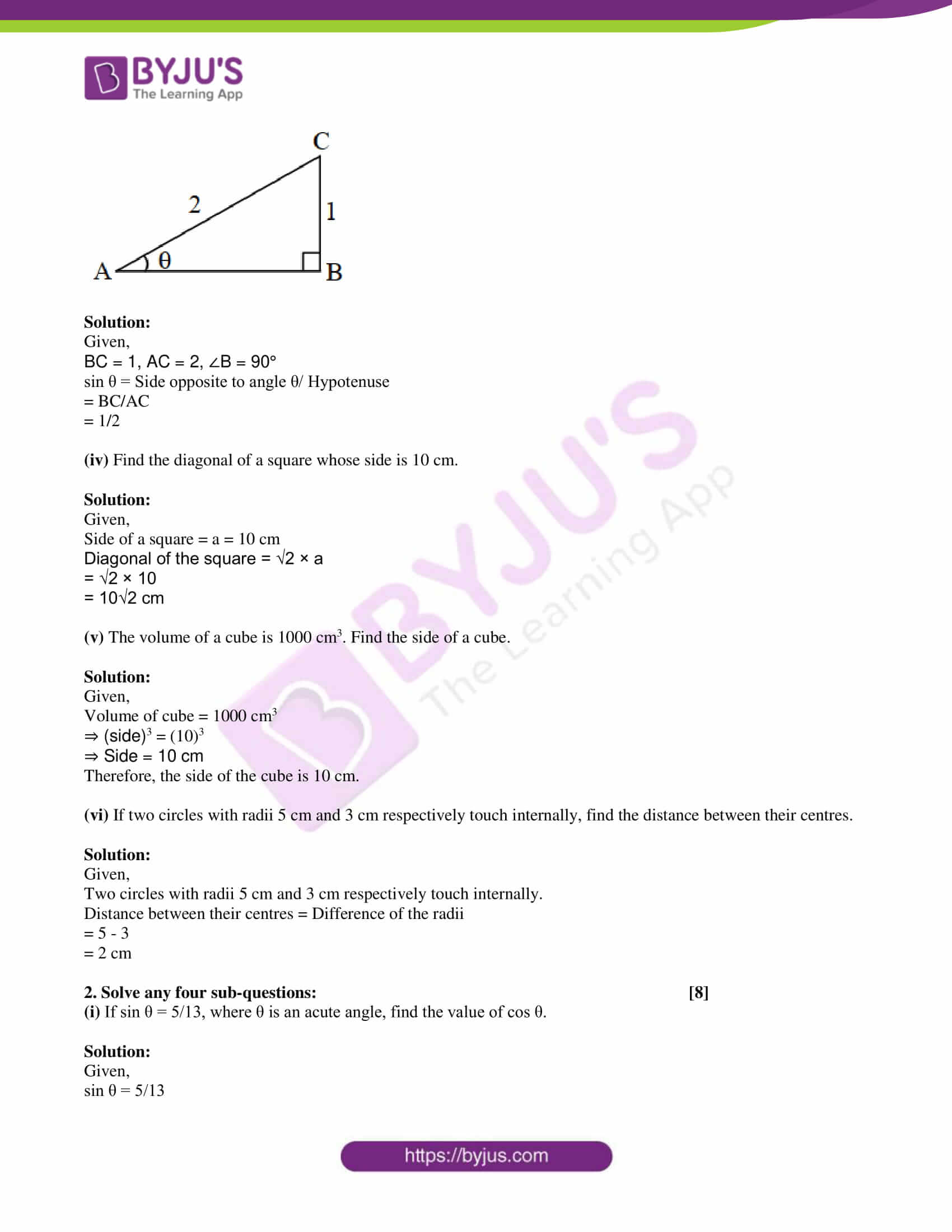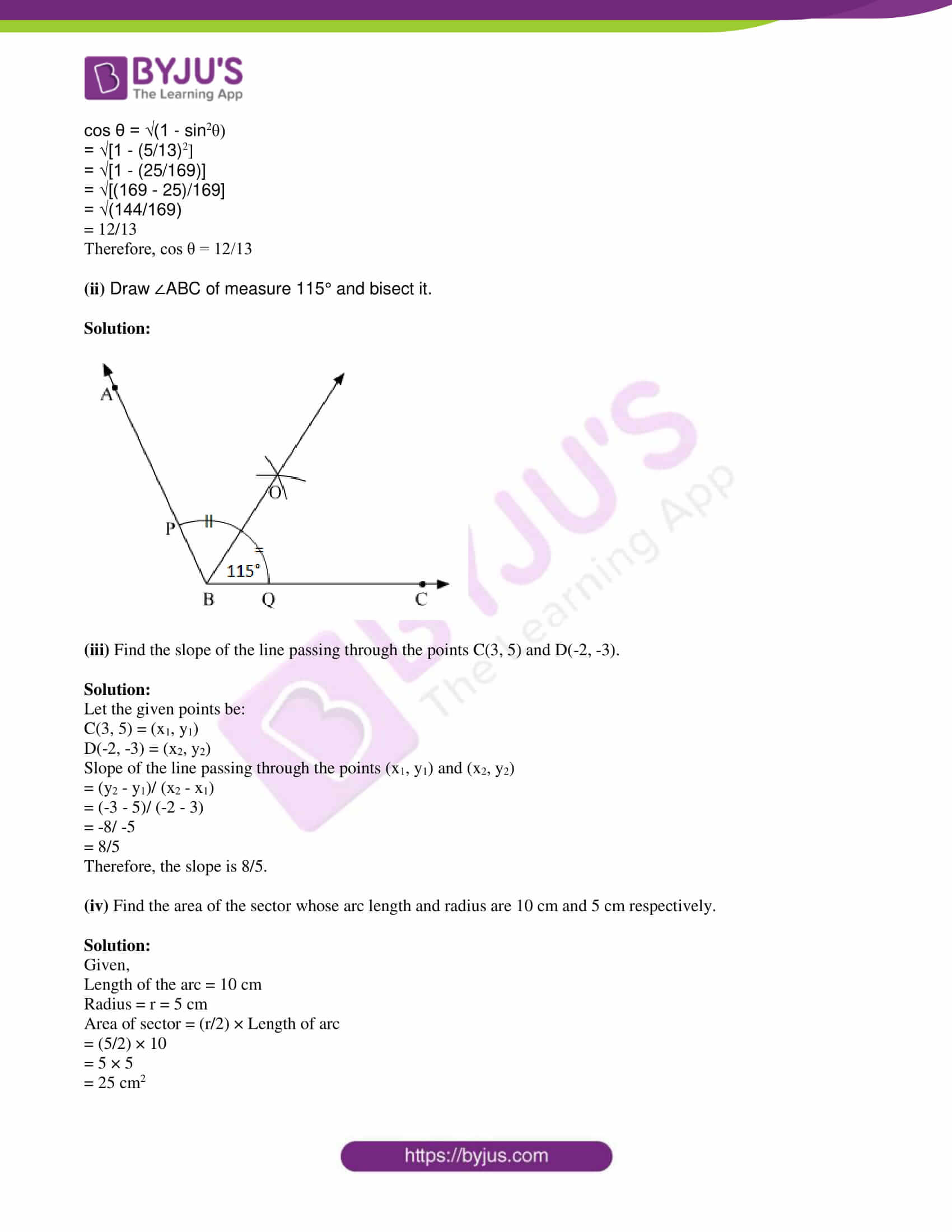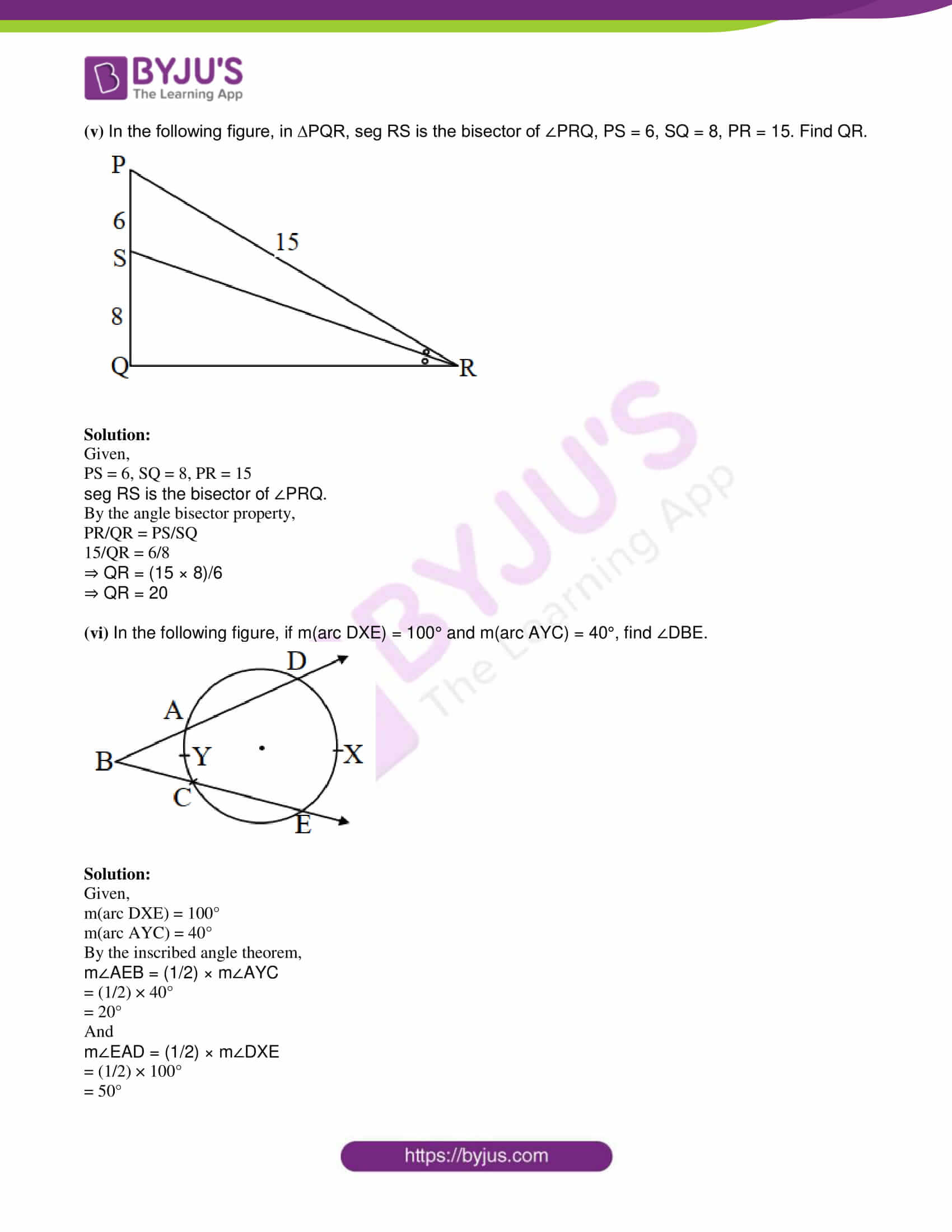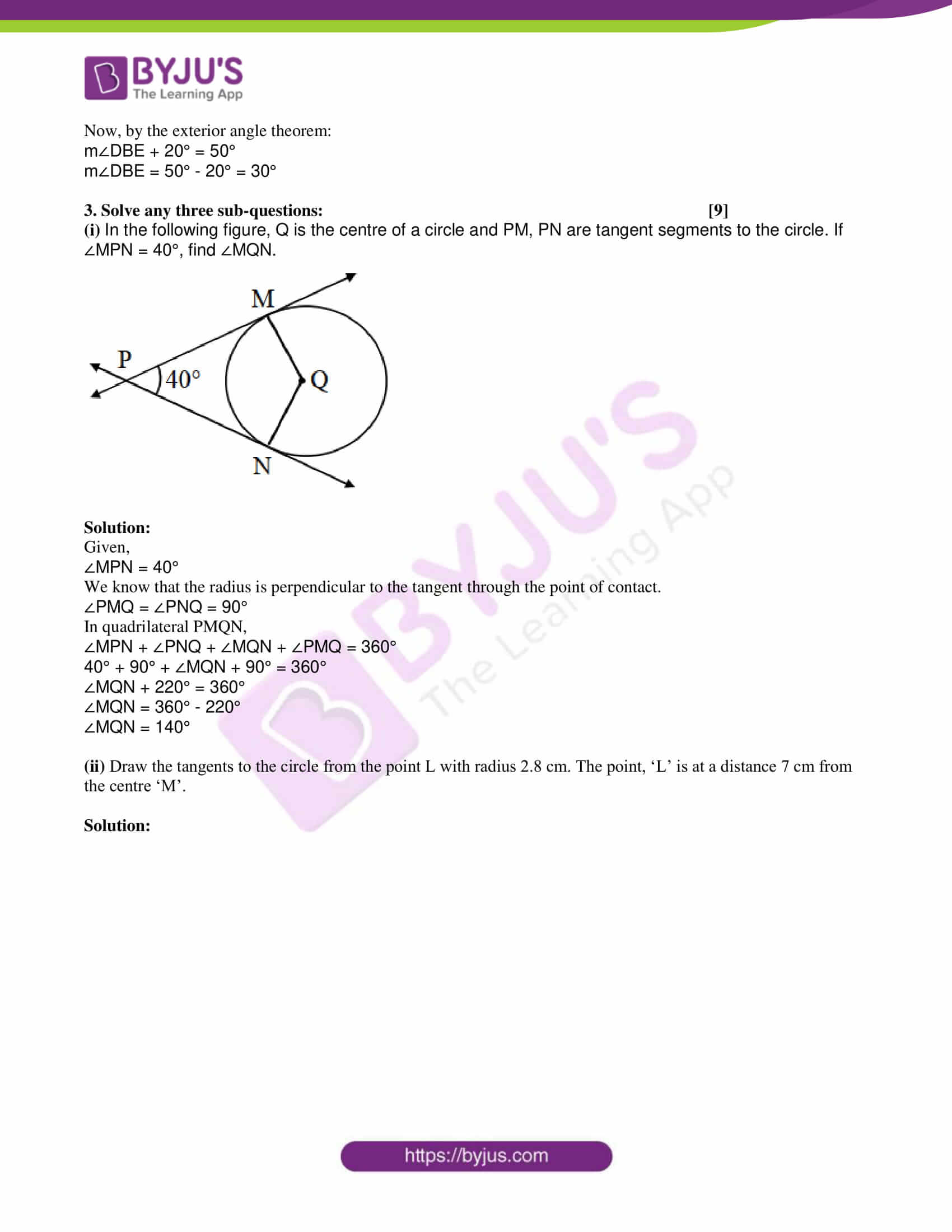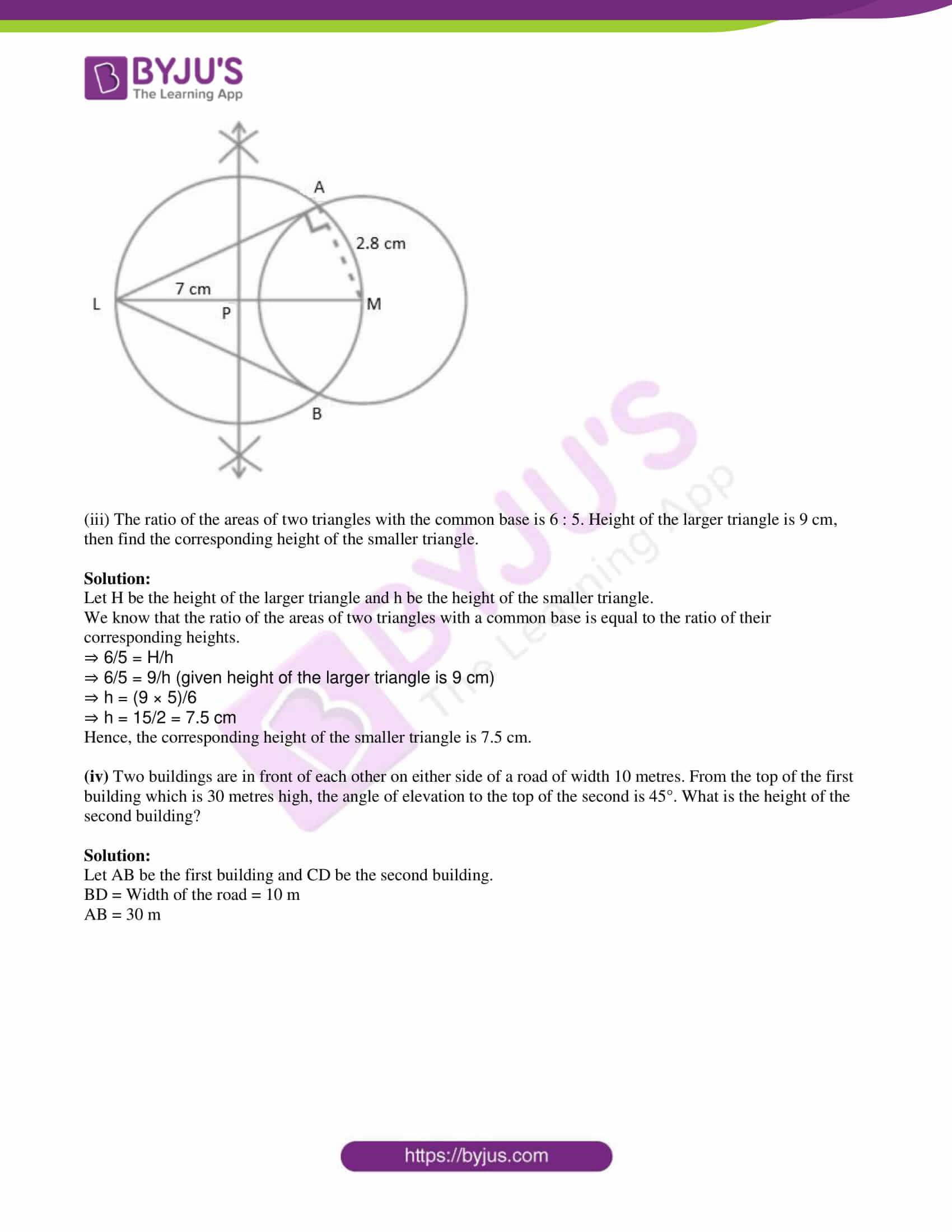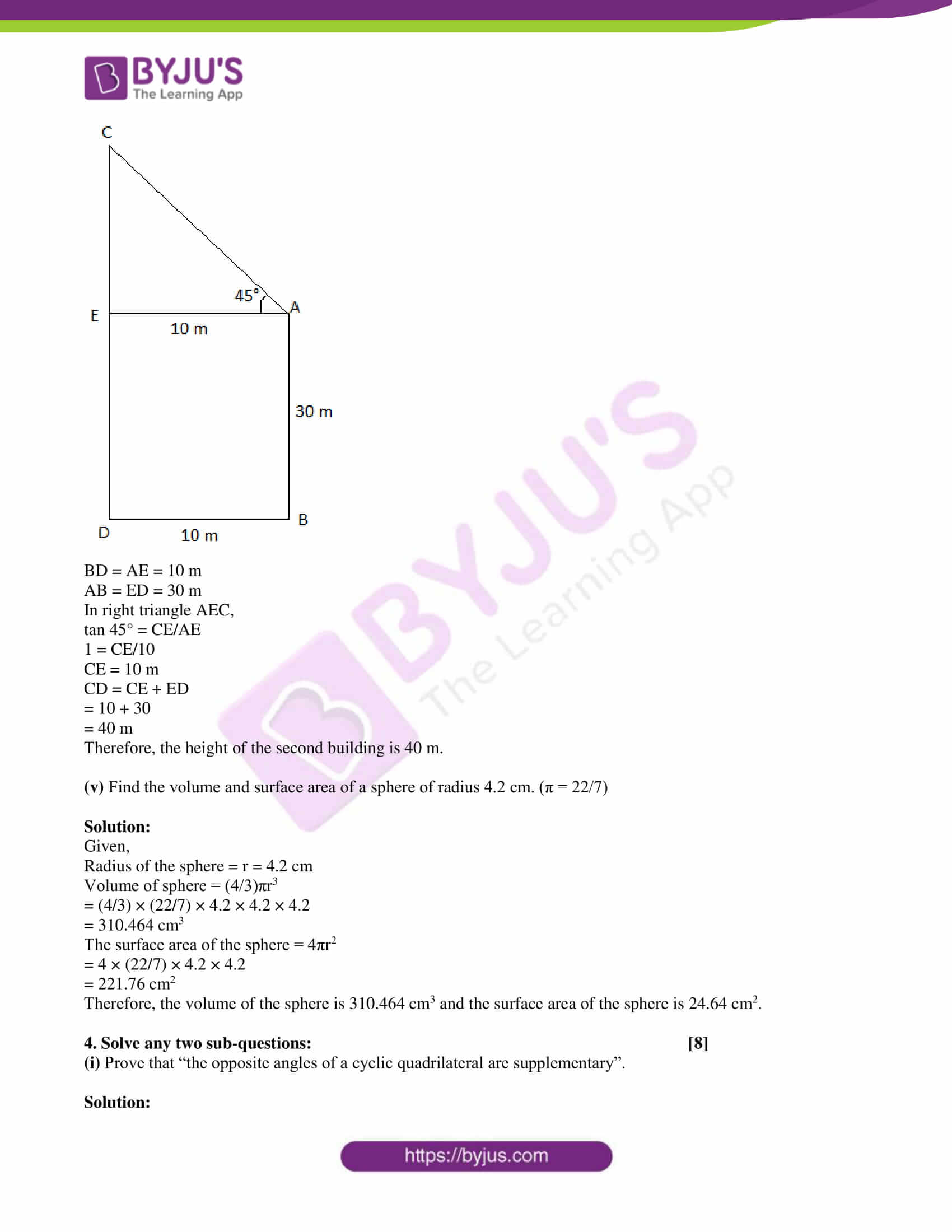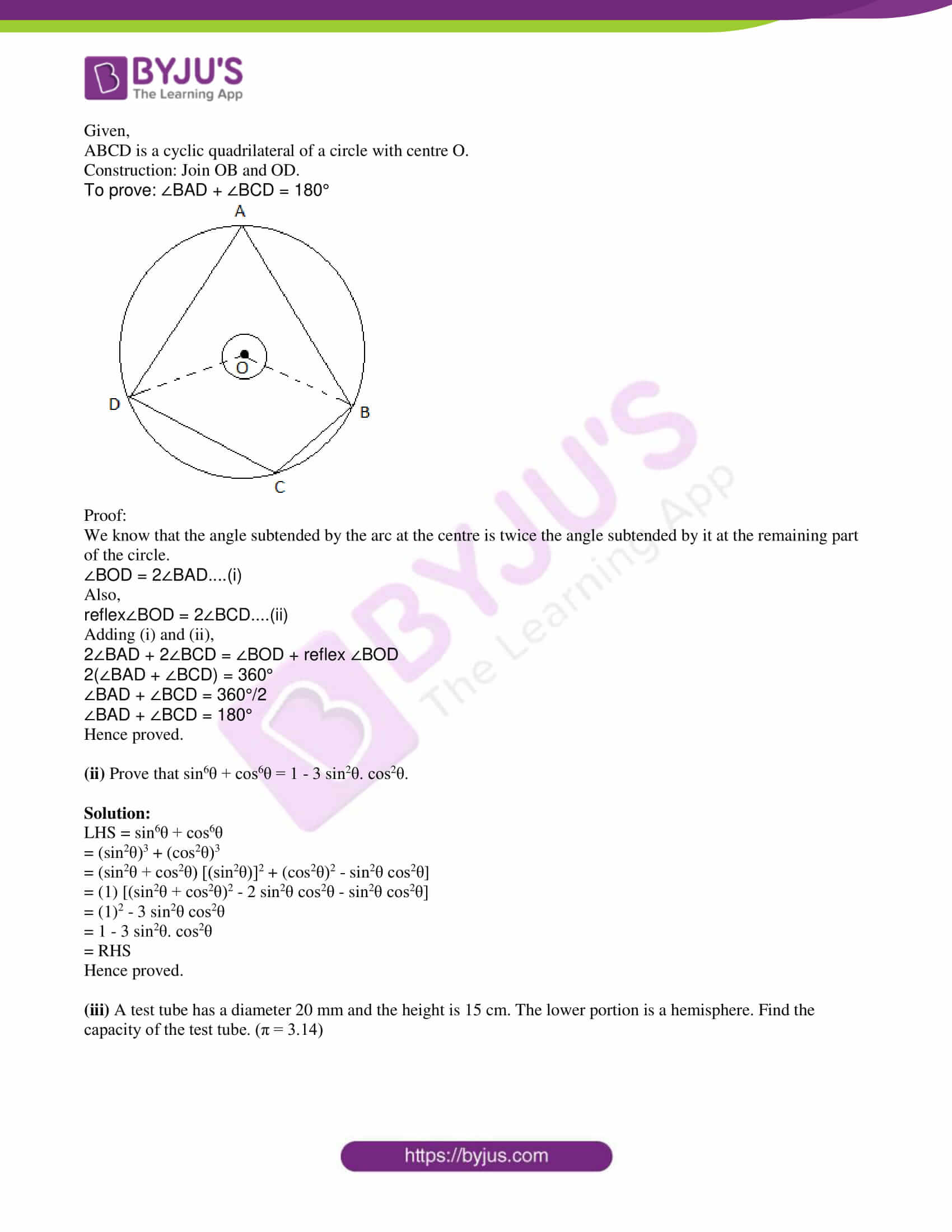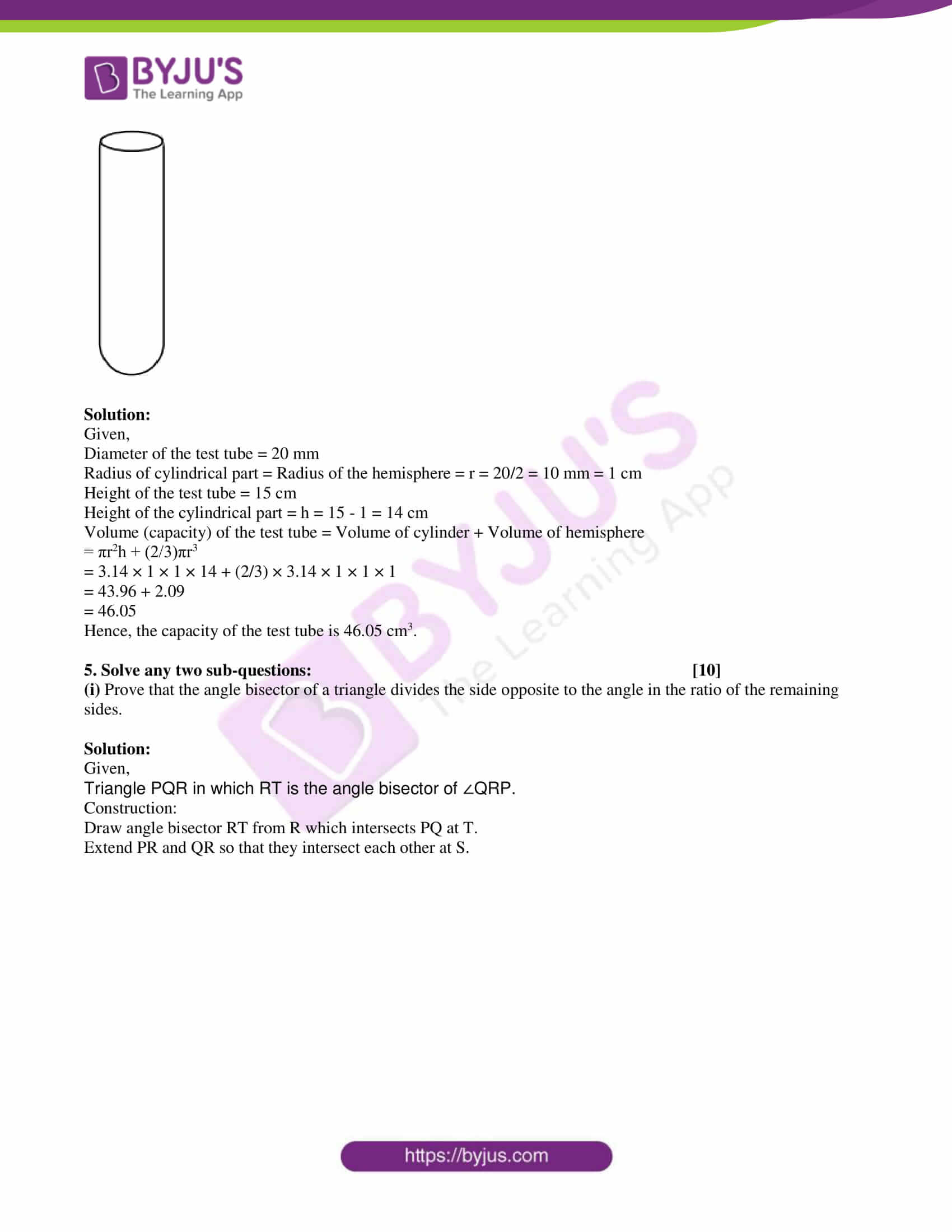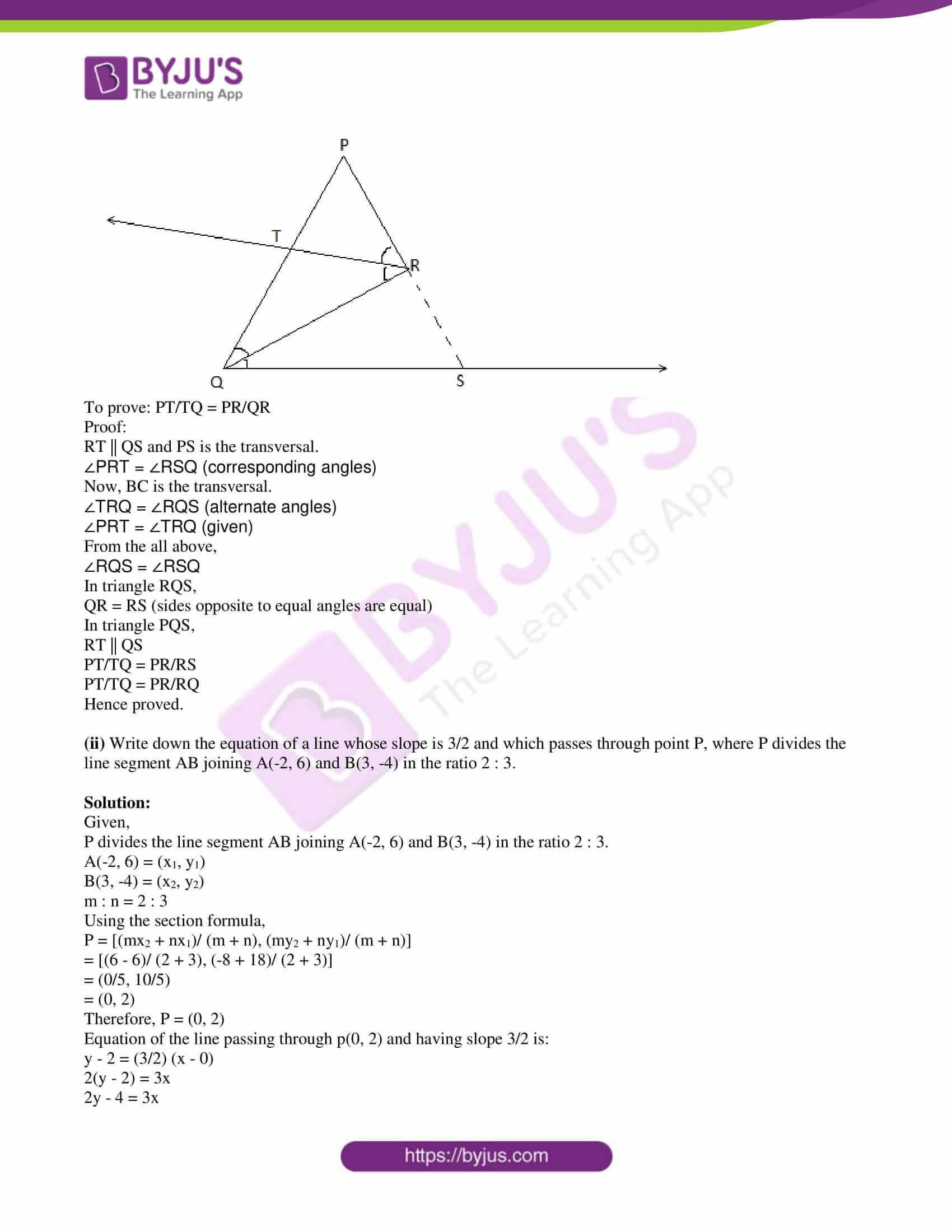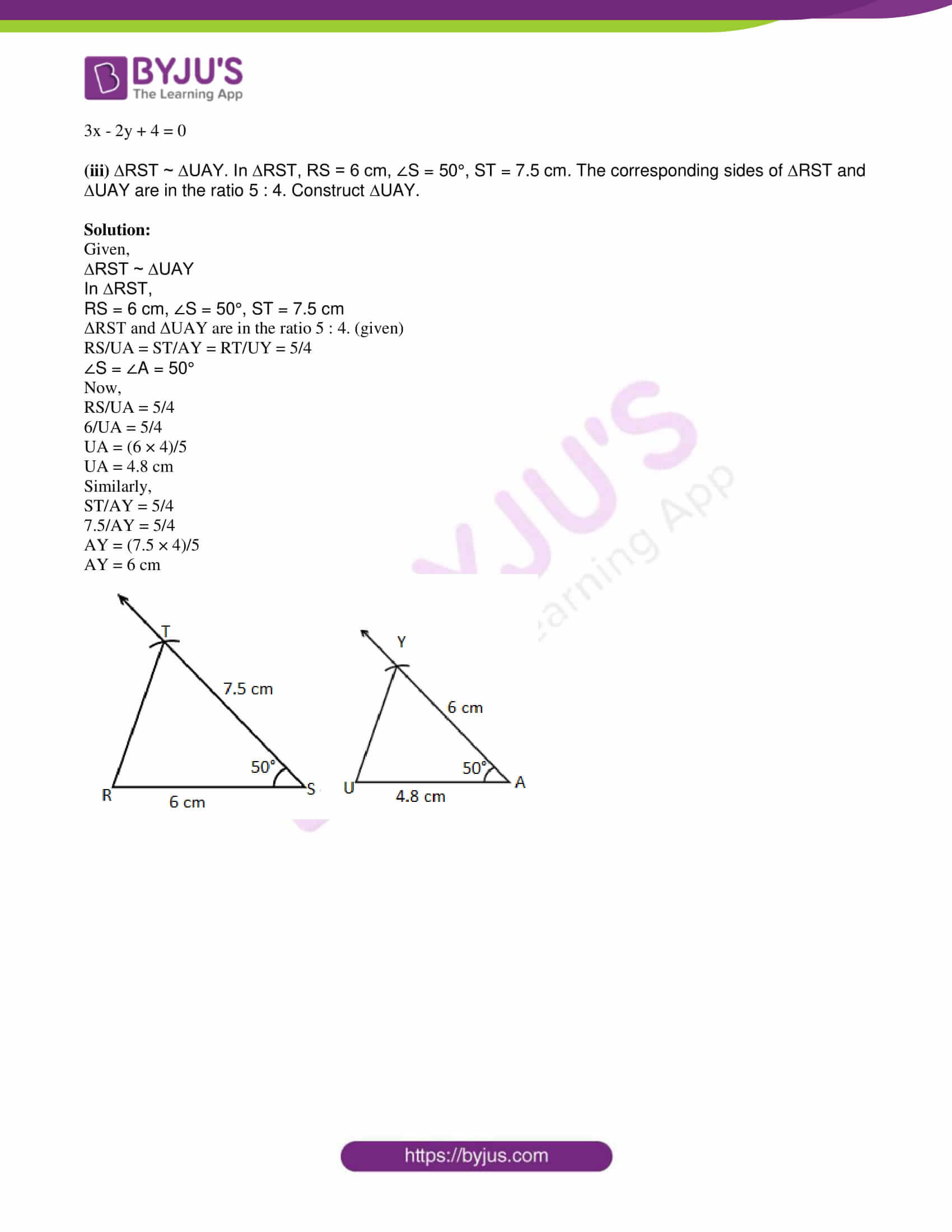PART – A

1. Solve any five sub-questions: 

(i) In the following figure, seg AB ⊥ seg BC, seg DC ⊥ seg BC. If AB = 2 and DC = 3, find A(∆ ABC)/ A(∆ DCB).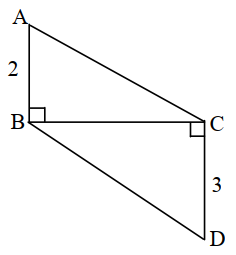Solution:

Given,

AB = 2 and DC = 3

We know that the ratio of areas of two triangles lying on the same base is equal to the ratio of their corresponding heights.

A(∆ ABC)/ A(∆ DCB) = AB/DC = 2/3

(ii) Find the slope and y-intercept of the line y = -2x + 3.

Solution:

Given,

y = -2x + 3

Comparing with the equation of line in slope-intercept form y = mx + c

Slope = m = -2

y-intercept = c = 3

(iii) In the following figure, in ∆ABC, BC = 1, AC = 2, ∠B = 90°. Find the value of sin θ.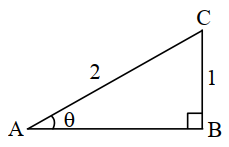Solution:

Given,

BC = 1, AC = 2, ∠B = 90°

sin θ = Side opposite to angle θ/ Hypotenuse

= BC/AC

= 1/2

(iv) Find the diagonal of a square whose side is 10 cm.

Solution:

Given,

Side of a square = a = 10 cm

Diagonal of the square = √2 × a

= √2 × 10

= 10√2 cm

(v) The volume of a cube is 1000 cm3. Find the side of a cube.

Solution:

Given,

Volume of cube = 1000 cm3

⇒ (side)3 = (10)3

⇒ Side = 10 cm

Therefore, the side of the cube is 10 cm.

(vi) If two circles with radii 5 cm and 3 cm respectively touch internally, find the distance between their centres.

Solution:

Given,

Two circles with radii 5 cm and 3 cm respectively touch internally.

Distance between their centres = Difference of the radii

= 5 – 3

= 2 cm

2. Solve any four sub-questions: 

(i) If sin θ = 5/13, where θ is an acute angle, find the value of cos θ.

Solution:

Given,

sin θ = 5/13

cos θ = √(1 – sin2θ)

= √[1 – (5/13)2]

= √[1 – (25/169)]

= √[(169 – 25)/169]

= √(144/169)

= 12/13

Therefore, cos θ = 12/13

(ii) Draw ∠ABC of measure 115° and bisect it.

Solution: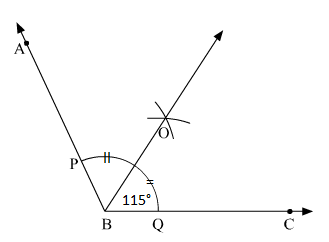(iii) Find the slope of the line passing through the points C(3, 5) and D(-2, -3).

Solution:

Let the given points be:

C(3, 5) = (x1, y1)

D(-2, -3) = (x2, y2)

Slope of the line passing through the points (x1, y1) and (x2, y2)

= (y2 – y1)/ (x2 – x1)

= (-3 – 5)/ (-2 – 3)

= -8/ -5

= 8/5

Therefore, the slope is 8/5.

(iv) Find the area of the sector whose arc length and radius are 10 cm and 5 cm, respectively.

Solution:

Given,

Length of the arc = 10 cm

Radius = r = 5 cm

Area of sector = (r/2) × Length of arc

= (5/2) × 10

= 5 × 5

= 25 cm2

(v) In the following figure, in ∆PQR, seg RS is the bisector of ∠PRQ, PS = 6, SQ = 8, PR = 15. Find QR.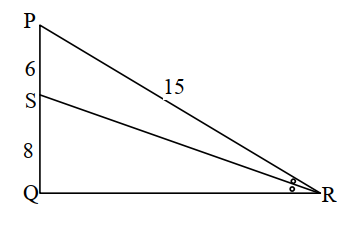Solution:

Given,

PS = 6, SQ = 8, PR = 15

seg RS is the bisector of ∠PRQ.

By the angle bisector property,

PR/QR = PS/SQ

15/QR = 6/8

⇒ QR = (15 × 8)/6

⇒ QR = 20

(vi) In the following figure, if m(arc DXE) = 100° and m(arc AYC) = 40°, find ∠DBE.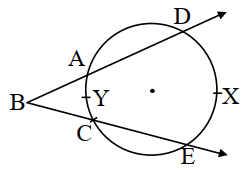Solution:

Given,

m(arc DXE) = 100°

m(arc AYC) = 40°

By the inscribed angle theorem,

m∠AEB = (1/2) × m∠AYC

= (1/2) × 40°

= 20°

And

= (1/2) × 100°

= 50°

Now, by the exterior angle theorem:

m∠DBE + 20° = 50°

m∠DBE = 50° – 20° = 30°

3. Solve any three sub-questions: 

(i) In the following figure, Q is the centre of a circle and PM, PN are tangent segments to the circle. If ∠MPN = 40°, find ∠MQN.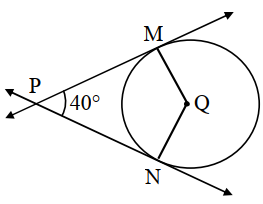Solution:

Given,

∠MPN = 40°

We know that the radius is perpendicular to the tangent through the point of contact.

∠PMQ = ∠PNQ = 90°

∠MPN + ∠PNQ + ∠MQN + ∠PMQ = 360°

40° + 90° + ∠MQN + 90° = 360°

∠MQN + 220° = 360°

∠MQN = 360° – 220°

∠MQN = 140°

(ii) Draw the tangents to the circle from the point L with radius 2.8 cm. The point, ‘L’ is at a distance 7 cm from the centre ‘M’.

Solution: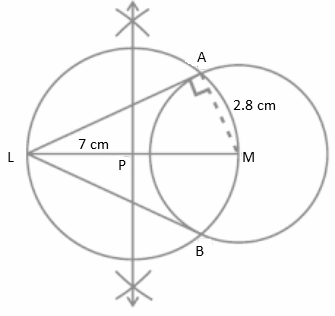(iii) The ratio of the areas of two triangles with the common base is 6 : 5. Height of the larger triangle is 9 cm, then find the corresponding height of the smaller triangle.

Solution:

Let H be the height of the larger triangle and h be the height of the smaller triangle.

We know that the ratio of the areas of two triangles with a common base is equal to the ratio of their corresponding heights.

⇒ 6/5 = H/h

⇒ 6/5 = 9/h (given height of the larger triangle is 9 cm)

⇒ h = (9 × 5)/6

⇒ h = 15/2 = 7.5 cm

Hence, the corresponding height of the smaller triangle is 7.5 cm.

(iv) Two buildings are in front of each other on either side of a road of width 10 metres. From the top of the first building which is 30 metres high, the angle of elevation to the top of the second is 45°. What is the height of the second building?

Solution:

Let AB be the first building and CD be the second building.

BD = Width of the road = 10 m

AB = 30 m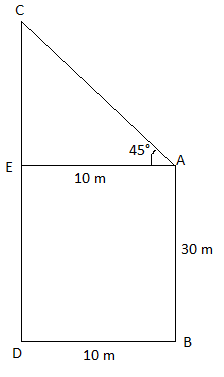BD = AE = 10 m

AB = ED = 30 m

In right triangle AEC,

tan 45° = CE/AE

1 = CE/10

CE = 10 m

CD = CE + ED

= 10 + 30

= 40 m

Therefore, the height of the second building is 40 m.

(v) Find the volume and surface area of a sphere of radius 4.2 cm. (π = 22/7)

Solution:

Given,

Radius of the sphere = r = 4.2 cm

Volume of sphere = (4/3)πr3

= (4/3) × (22/7) × 4.2 × 4.2 × 4.2

= 310.464 cm3

The surface area of the sphere = 4πr2

= 4 × (22/7) × 4.2 × 4.2

= 221.76 cm2

Therefore, the volume of the sphere is 310.464 cm3 and the surface area of the sphere is 24.64 cm2.

4. Solve any two sub-questions: 

(i) Prove that “the opposite angles of a cyclic quadrilateral are supplementary”.

Solution:

Given,

ABCD is a cyclic quadrilateral of a circle with centre O.

Construction: Join OB and OD.

To prove: ∠BAD + ∠BCD = 180°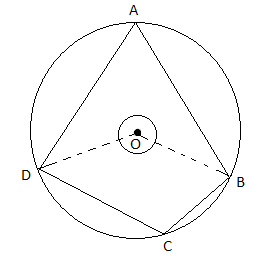Proof:

We know that the angle subtended by the arc at the centre is twice the angle subtended by it at the remaining part of the circle.

Also,

reflex∠BOD = 2∠BCD….(ii)

2∠BAD + 2∠BCD = ∠BOD + reflex ∠BOD

Hence proved.

(ii) Prove that sin6θ + cos6θ = 1 – 3 sin2θ. cos2θ.

Solution:

LHS = sin6θ + cos6θ

= (sin2θ)3 + (cos2θ)3

= (sin2θ + cos2θ) [(sin2θ)]2 + (cos2θ)2 – sin2θ cos2θ]

= (1) [(sin2θ + cos2θ)2 – 2 sin2θ cos2θ – sin2θ cos2θ]

= (1)2 – 3 sin2θ cos2θ

= 1 – 3 sin2θ. cos2θ

= RHS

Hence proved.

(iii) A test tube has a diameter 20 mm and the height is 15 cm. The lower portion is a hemisphere. Find the capacity of the test tube. (π = 3.14)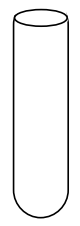Solution:

Given,

Diameter of the test tube = 20 mm

Radius of cylindrical part = Radius of the hemisphere = r = 20/2 = 10 mm = 1 cm

Height of the test tube = 15 cm

Height of the cylindrical part = h = 15 – 1 = 14 cm

Volume (capacity) of the test tube = Volume of cylinder + Volume of hemisphere

= πr2h + (2/3)πr3

= 3.14 × 1 × 1 × 14 + (2/3) × 3.14 × 1 × 1 × 1

= 43.96 + 2.09

= 46.05

Hence, the capacity of the test tube is 46.05 cm3.

5. Solve any two sub-questions: 

(i) Prove that the angle bisector of a triangle divides the side opposite to the angle in the ratio of the remaining sides.

Solution:

Given,

Triangle PQR in which RT is the angle bisector of ∠QRP.

Construction:

Draw angle bisector RT from R which intersects PQ at T.

Extend PR and QR so that they intersect each other at S.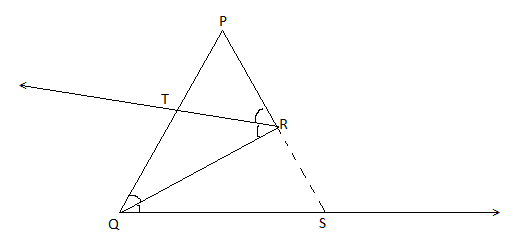To prove: PT/TQ = PR/QR

Proof:

RT || QS and PS is the transversal.

∠PRT = ∠RSQ (corresponding angles)

Now, BC is the transversal.

∠TRQ = ∠RQS (alternate angles)

∠PRT = ∠TRQ (given)

From the all above,

∠RQS = ∠RSQ

In triangle RQS,

QR = RS (sides opposite to equal angles are equal)

In triangle PQS,

RT || QS

PT/TQ = PR/RS

PT/TQ = PR/RQ

Hence proved.

(ii) Write down the equation of a line whose slope is 3/2 and which passes through point P, where P divides the line segment AB joining A(-2, 6) and B(3, -4) in the ratio 2 : 3.

Solution:

Given,

P divides the line segment AB joining A(-2, 6) and B(3, -4) in the ratio 2 : 3.

A(-2, 6) = (x1, y1)

B(3, -4) = (x2, y2)

m : n = 2 : 3

Using the section formula,

P = [(mx2 + nx1)/ (m + n), (my2 + ny1)/ (m + n)]

= [(6 – 6)/ (2 + 3), (-8 + 18)/ (2 + 3)]

= (0/5, 10/5)

= (0, 2)

Therefore, P = (0, 2)

Equation of the line passing through p(0, 2) and having slope 3/2 is:

y – 2 = (3/2) (x – 0)

2(y – 2) = 3x

2y – 4 = 3x

3x – 2y + 4 = 0

(iii) ∆RST ~ ∆UAY. In ∆RST, RS = 6 cm, ∠S = 50°, ST = 7.5 cm. The corresponding sides of ∆RST and ∆UAY are in the ratio 5 : 4. Construct ∆UAY.

Solution:

Given,

∆RST ~ ∆UAY

In ∆RST,

RS = 6 cm, ∠S = 50°, ST = 7.5 cm

ΔRST and ΔUAY are in the ratio 5 : 4. (given)

RS/UA = ST/AY = RT/UY = 5/4

∠S = ∠A = 50°

Now,

RS/UA = 5/4

6/UA = 5/4

UA = (6 × 4)/5

UA = 4.8 cm

Similarly,

ST/AY = 5/4

7.5/AY = 5/4

AY = (7.5 × 4)/5

AY = 6 cm